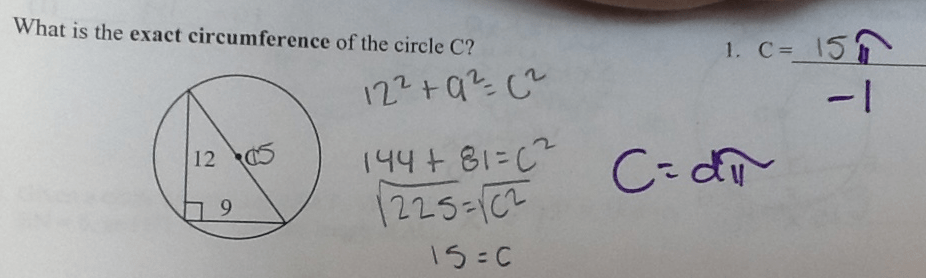Categories

# Circumference and Right TrianglesSay something smart in the comments about why kids forget “little” things on problems.  Or, alternatively, disagree with the premise of my first question. (How’s that for a lousy prompt?)

Thanks to Mark Kingsbury for the submission!

## 4 replies on “Circumference and Right Triangles”0112358says:

I think it’s possible that the student was misled by the use of the letter “C” in this problem. The text of the question indicates that the circle itself is called C, i.e. C = Circle, but I think it’s unlikely that this caused the student’s error.

The answer space in the top right has “C =” printed there, which suggests C = Circumference (which I assume was the actual intention of the test setter).

In the diagram, the “C” is labeling the centre of the circle (again suggesting C = Circle), but unfortunately, it is also positioned right next to the line indicating the diameter (suggesting C = Diameter, although this would hopefully strike a student as being unconventional notation).

Finally, Pythagoras’ Theorem is often expressed as a^2 + b^2 = c^2, and so it’s possible that the student made the association C = Hypothenuse, also suggested by the diagram.

The fact that the student wrote “15” over the C printed on the diagram to me indicates that the student was making the association of C = Diameter or C = Hypothenuse.

That said, “exact circumference” was highlighted in bold, so the student didn’t read the prompt text very well (or at all). It’s possible that the student was distracted/emboldened by identifying a calculation they could easily do (a^2 + b^2 = c^2), and so rushed to compute it without actually reading the question, but that’s pure speculation.

My students do something similar. When a question is multi-parted like this, they will do all the “hard work” of getting the first part and then forget to finish the actual problem. Giving the student two slots for answers or asking both questions might help. Part A: What is the diameter? Part B: What is the circumference? I guess that might be leading the student on when you want them to understand the connection on their own, but it could help eliminate this problem. Or set these up in two separate problems. Do you want to test their understanding of Pythagorean Theorem to find diameter? Or do you want to test their understanding of how circumference is related to diameter? Or do you actually want to test their synthesis skills of putting these together? I think the student has only failed on the last goal here.

I don’t know if it’s smart, but I’ll say often kids forget the little things because they are lazy and in a rush. They think, “ya, ya, I know this, whatever, next problem” so they can be done as fast as possible and on to things they’d rather be doing.

Multipart questions necessarily slow them down and put in all the prompts that make sure they aren’t skipping little things from rushing.

I truly think that the problem here is the mixup of C between the Pythagorean Theorem and circumference. I know with my kids, they always use a^2 + b^2 = c^2, even if the side they are solving for is already labeled with a different variable. I went to a session about graphic organizers recently, and there was a good one that was meant to explain the variables in different formulas because they are often the same letter. I’ve found that I really have to push my students to explain to me what each variable represents, rather than just plugging away blindly.